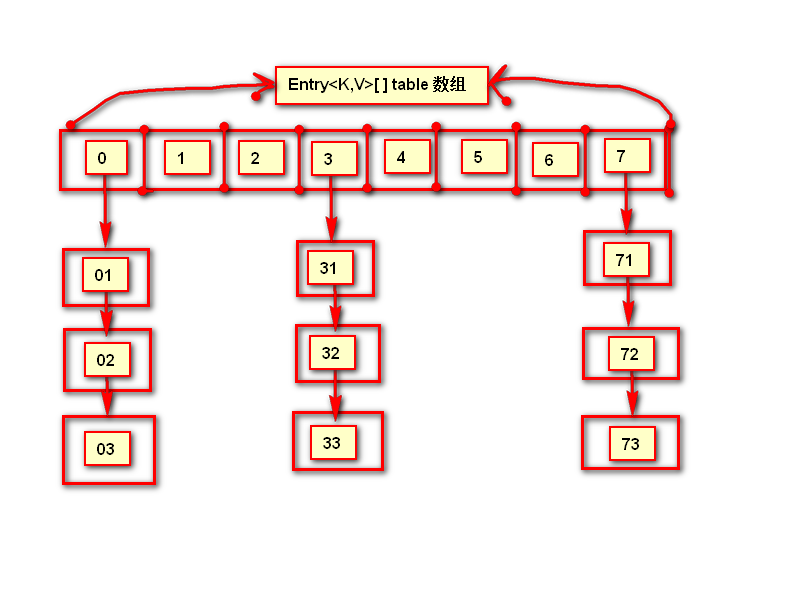# equals和hashCode源码解析

2015/02/07 10:45

equals和hashCode网上也有很多的资料。这里只是记录下我目前的理解与认识。

public native int hashCode();
public boolean equals(Object obj) {
return (this == obj);
}

/**
* 关注点1
* Returns a hash code value for the object. This method is
* supported for the benefit of hash tables such as those provided by
* <p>
* The general contract of {@code hashCode} is:
* <ul>
* <li>Whenever it is invoked on the same object more than once during
*     an execution of a Java application, the {@code hashCode} method
*     must consistently return the same integer, provided no information
*     used in {@code equals} comparisons on the object is modified.
*     This integer need not remain consistent from one execution of an
*     application to another execution of the same application.
* 关注点2
* <li>If two objects are equal according to the {@code equals(Object)}
*     method, then calling the {@code hashCode} method on each of
*     the two objects must produce the same integer result.
* <li>It is <em>not</em> required that if two objects are unequal
*     according to the {@link java.lang.Object#equals(java.lang.Object)}
*     method, then calling the {@code hashCode} method on each of the
*     two objects must produce distinct integer results.  However, the
*     programmer should be aware that producing distinct integer results
*     for unequal objects may improve the performance of hash tables.
* </ul>
* <p>
* 关注点3
* As much as is reasonably practical, the hashCode method defined by
* class {@code Object} does return distinct integers for distinct
* objects. (This is typically implemented by converting the internal
* address of the object into an integer, but this implementation
* technique is not required by the
* Java<font size="-2"><sup>TM</sup></font> programming language.)
*
* @return  a hash code value for this object.
* @see     java.lang.Object#equals(java.lang.Object)
* @see     java.lang.System#identityHashCode
*/
public native int hashCode();

java世界里的相同：

public class Person {

private String name;
private int age;

public String getName() {
return name;
}
public void setName(String name) {
this.name = name;
}
public int getAge() {
return age;
}
public void setAge(int age) {
this.age = age;
}
@Override
public boolean equals(Object obj) {
if(!(obj instanceof Person)){
return false;
}
Person tmp=(Person)obj;
return name.equals(tmp.getName()) && age==tmp.getAge();
}

}

static final Entry<?,?>[] EMPTY_TABLE = {};
transient Entry<K,V>[] table = (Entry<K,V>[]) EMPTY_TABLE;

HashMap内部是由Entry<K,V>类型的数组table来存储数据的。来看下Entry<K,V>的代码：
static class Entry<K,V> implements Map.Entry<K,V> {
final K key;
V value;
Entry<K,V> next;
int hash;

/**
* Creates new entry.
*/
Entry(int h, K k, V v, Entry<K,V> n) {
value = v;
next = n;
key = k;
hash = h;
}
//略
}

Entry<K,V>有四个重要的属性，是一对key和value的结合，同时包含下一个Entry<K,V>，就像链表一样，最后一个就是哈希值h（这个哈希值就是key的hashCode方法的返回值经过hash运算得到的值）。public V put(K key, V value) {
if (table == EMPTY_TABLE) {
inflateTable(threshold);
}
if (key == null)
return putForNullKey(value);
int hash = hash(key);
int i = indexFor(hash, table.length);
for (Entry<K,V> e = table[i]; e != null; e = e.next) {
Object k;
if (e.hash == hash && ((k = e.key) == key || key.equals(k))) {
V oldValue = e.value;
e.value = value;
e.recordAccess(this);
return oldValue;
}
}

modCount++;
return null;
}

final int hash(Object k) {
int h = hashSeed;
if (0 != h && k instanceof String) {
return sun.misc.Hashing.stringHash32((String) k);
}

h ^= k.hashCode();

// This function ensures that hashCodes that differ only by
// constant multiples at each bit position have a bounded
// number of collisions (approximately 8 at default load factor).
h ^= (h >>> 20) ^ (h >>> 12);
return h ^ (h >>> 7) ^ (h >>> 4);
}

static int indexFor(int h, int length) {
return h & (length-1);
}

HashMap为什么要多引入key的hash是否一致的判断条件呢？为什么不仅仅判断equals方法是否一样？

hashCode的重写的原则：当equals方法返回true，则两个对象的hashCode必须一样。

public V get(Object key) {
if (key == null)
return getForNullKey();
Entry<K,V> entry = getEntry(key);

return null == entry ? null : entry.getValue();
}

final Entry<K,V> getEntry(Object key) {
if (size == 0) {
return null;
}

int hash = (key == null) ? 0 : hash(key);
for (Entry<K,V> e = table[indexFor(hash, table.length)];
e != null;
e = e.next) {
Object k;
if (e.hash == hash &&
((k = e.key) == key || (key != null && key.equals(k))))
return e;
}
return null;
}

hash&length-1结果相同我们称为冲突

Set与List相比是无序的，不允许元素重复。元素重复的依据和HashMap对key的要求是一样的。即所存元素的hash值一样并且equals相同才是一样的元素。看下代码：

private transient HashMap<E,Object> map;

private static final Object PRESENT = new Object();

public boolean add(E e) {
return map.put(e, PRESENT)==null;
}### 作者的其它热门文章

0
6 收藏

1 评论
6 收藏
0